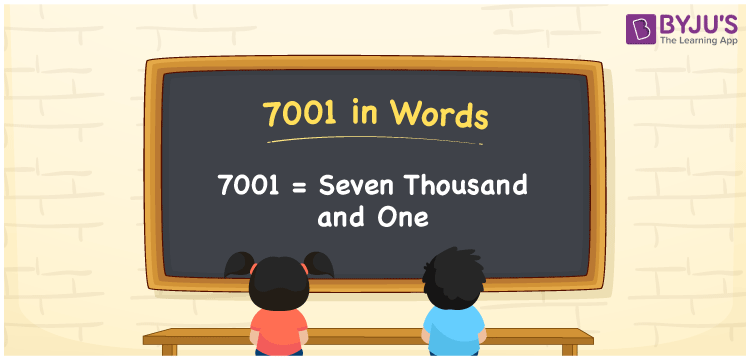# 7001 in Words

7001 in words is written as Seven thousand one. In both the International System of Numerals and the Indian System of Numerals, 7001 is written as Seven thousand one. The number 7001 is a Cardinal Number as it could represent some quantity. For example, “the speakers cost 7001 rupees”.

 7001 in Words Seven thousand one Seven thousand one in Number 7001

## 7001 in English Words

7001 in English words is read as “Seven thousand one”.## How to Write 7001 in Words?

To write 7001 in words, we shall use the place value chart. In the place value chart, put 7 in the thousands, 0 in the hundreds and tens, and 1 in the ones, respectively. Let us make a place value chart to write the number 7001 in words.

 Thousands Hundreds Tens Ones 7 0 0 1

Thus, we can write the expanded form as

7 × Thousand + 0 × Hundred + 0 × Ten + 1 × One

= 7 × 1000 + 0 × 100 + 0 × 10 + 1 × 1

= 7000 + 0 + 0 + 1

= 7001

= Seven thousand one.

7001 is a natural number, the successor of 7000 and the predecessor of 7002.

7001 in words – Seven thousand one

• Is 7001 an odd number? – Yes
• Is 7001 an even number? – No
• Is 7001 a perfect square number? – No
• Is 7001 a perfect cube number? – No
• Is 7001 a prime number? – Yes
• Is 7001 a composite number? – No

## Frequently Asked Questions on 7001 in Words

Q1

### How to write 7001 in words?

7001 in words is written as Seven thousand one.
Q2

### How to write 7001 in the International and Indian System of Numerals?

In both, the system of numerals, 7001 in words, is written as Seven thousand one.
Q3

### How to write 7001 in a place value chart?

In the place value chart, write 7 in the thousands, 0 in the hundreds and tens, and 1 in the ones, respectively.